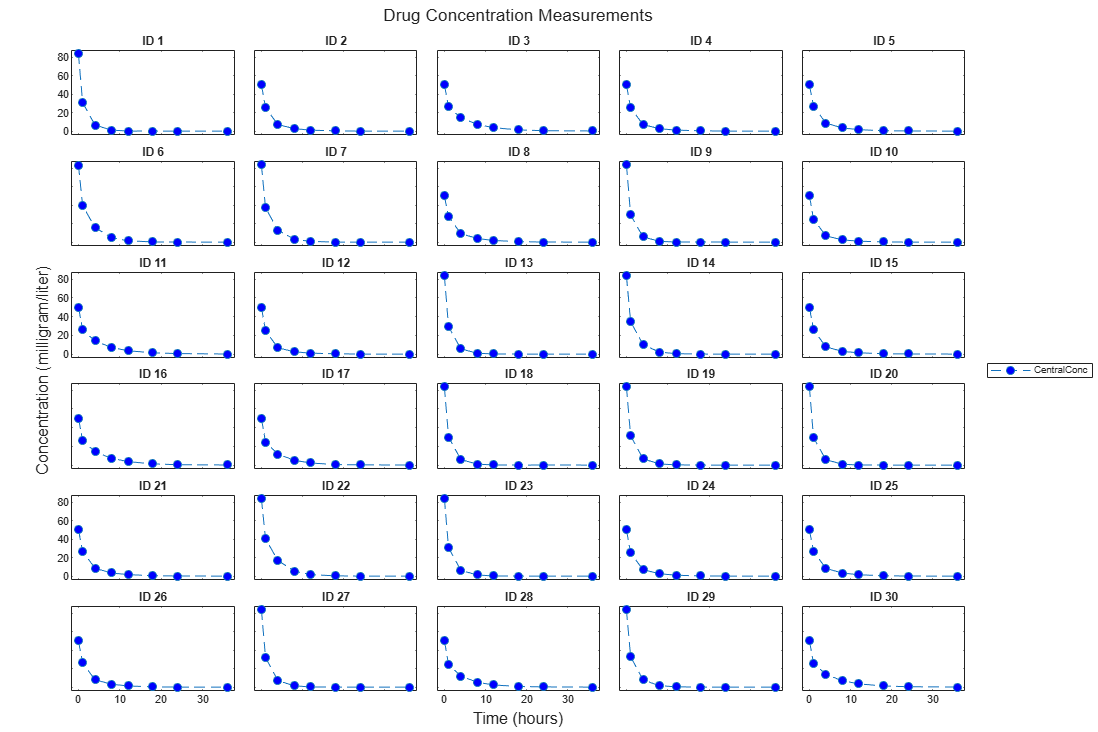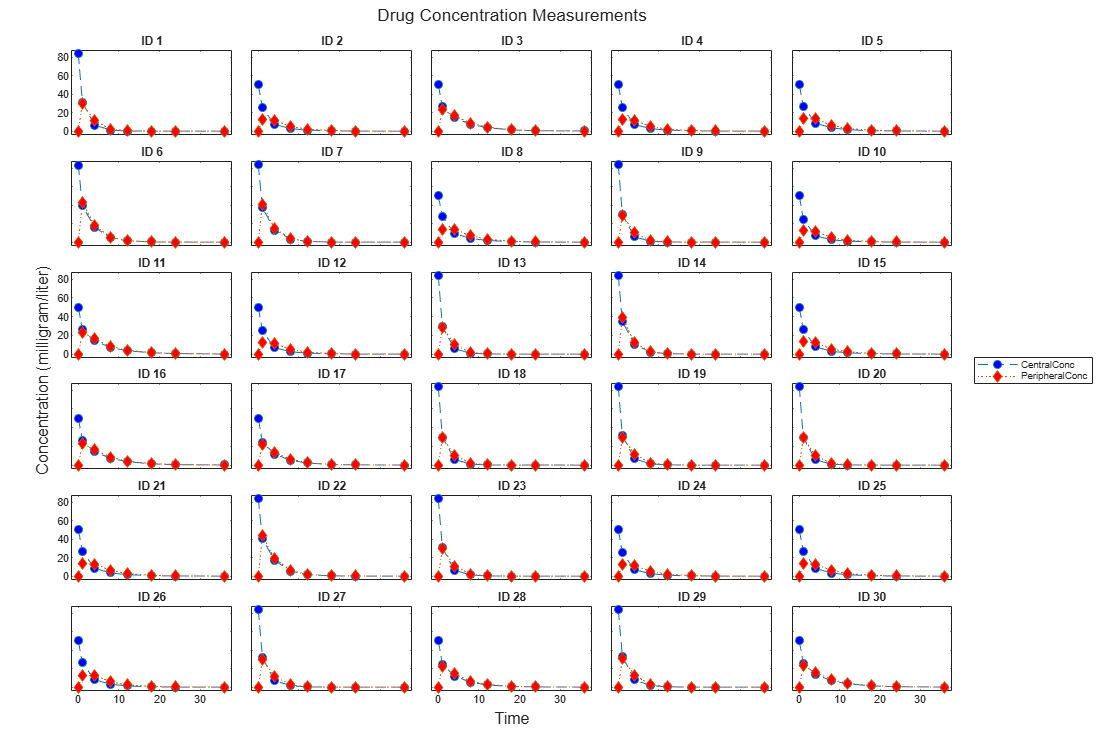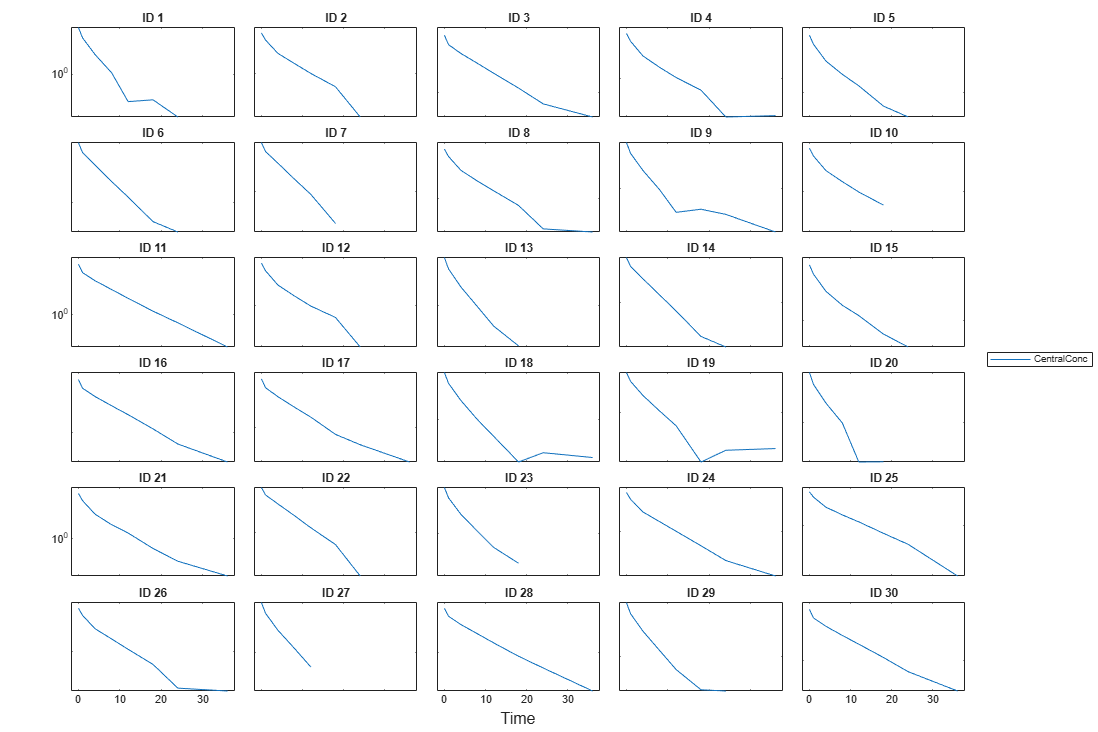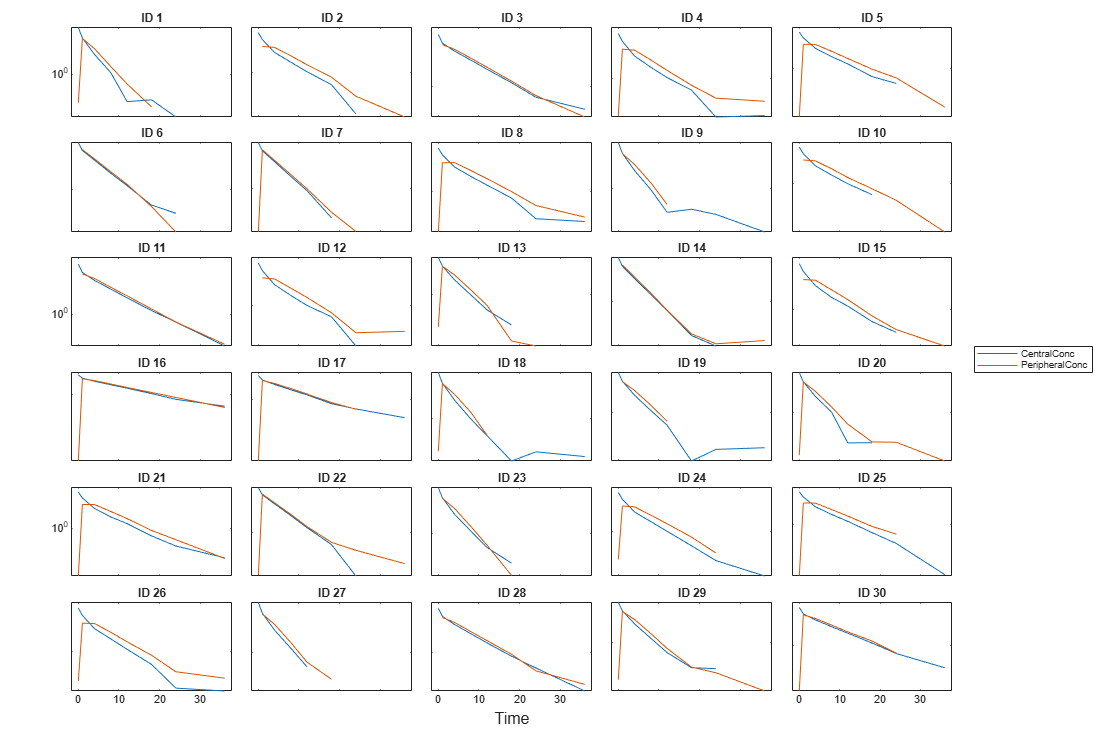# sbiotrellis

Plot data or simulation results in trellis plot

## Syntax

``trellisplot = sbiotrellis(data,groupCol,xCol,yCol)``
``trellisplot = sbiotrellis(data,groupCol,xCol,yCol,Name,Value)``
``trellisplot = sbiotrellis(data,fcnHandle,groupCol,xCol,yCol)``
``trellisplot = sbiotrellis(simData,fcnHandle,xCol,yCol)``

## Description

example

````trellisplot = sbiotrellis(data,groupCol,xCol,yCol)` plots each group in `data` as defined by the group column variable `groupCol` into its own subplot. The data defined by column `xCol` is plotted against the data defined by column(s) `yCol`.```

example

````trellisplot = sbiotrellis(data,groupCol,xCol,yCol,Name,Value)` uses additional options specified by one or more `Name,Value` pair arguments that are supported by the `plot` command.```

example

````trellisplot = sbiotrellis(data,fcnHandle,groupCol,xCol,yCol)` plots each group in `data` as defined by the group column variable `groupCol` into its own subplot. `sbiotrellis` creates the subplot by calling the function handle, `fcnHandle`, with input arguments defined by the `data` columns `xCol` and `yCol`. The `fcnHandle` cannot be empty and must be specified.The `fcnHandle` must have the signature `fcnHandle(x,y)`, where `x` is a numeric column vector, and `y` is a matrix with the same number of rows as `x`. For instance, if you want to create a trellis plot with a logarithmic y-axis, use `@semilogy` as the function handle, where `semilogy` is the function that plots data with logarithmic scale for the y-axis.```

example

````trellisplot = sbiotrellis(simData,fcnHandle,xCol,yCol)` plots each group in `simData` into its own subplot. `sbiotrellis` creates the subplot by calling the function handle, `fcnHandle` with input arguments defined by the columns `xCol` and `yCol`. The `fcnHandle` can be empty (`''` or `[]`). If empty, the default time plot is created using the handle `@plot`.The `fcnHandle` must have the signature `fcnHandle(simDataI,xCol,yCol)`, where `simDataI` is a single `SimData` object, and `xCol` and `yCol` are the corresponding input arguments to `sbiotrellis`. TipUse the `plot` method of a sbiotrellis object to overlay a `SimData` object or a `dataset` on an existing `sbiotrellis` plot. For example, `plot(trellisplot,...)` adds a plot to the object `trellisplot`. The `SimData` or `dataset` that is being plotted must have the same number of elements or groups as the `trellisplot` object. The `plot` method has the same input arguments as `sbiotrellis`. ```

## Examples

collapse all

Load a sample dataset. The data contains measurements of drug concentration in the central and peripheral compartments for 30 subjects.

`load('sd5_302RAgeSex.mat');`

Create a trellis plot of the Central concentrations for each subject.

```t = sbiotrellis(data, 'ID', 'Time', 'CentralConc',... 'Marker', 'o','LineStyle','--','MarkerFaceColor','b'); % Resize the figure. t.hFig.Position(:) = [100 100 1200 800];```Use the `plot` method of the sbiotrellis object to overlay the peripheral concentration data on the same plot.

```plot(t,data,'ID','Time','PeripheralConc','Marker','d',... 'LineStyle',':','MarkerFaceColor','r');```Specify the function handle `@semilogy` to change the y-axis to log scale.

```t2 = sbiotrellis(data,@semilogy,'ID','Time','CentralConc'); %Resize the figure t2.hFig.Position(:) = [100 100 1200 800];````plot(t2,data,@semilogy,'ID','Time','PeripheralConc');`## Input Arguments

collapse all

Data, specified as a `dataset` (Statistics and Machine Learning Toolbox) containing grouped data, a ```groupedData object```, or a `table`.

Group column name, specified as a character vector or string which is the name of a column in `data` that contains grouping information or an empty name `''` or `""`which implies there is only one group in `data`.

Name of a column to plot on the x-axis, specified as a character vector or string.

If `data` is `groupedData`, then `xCol` can also be an empty name `''` or `""`, and the x-coordinates of the data are determined by the variable specified in `DATA.Properties.IndependentVariableName`.

If `data` is `dataset` (Statistics and Machine Learning Toolbox) or `table`, then `xCol` cannot be empty.

Name of a column to plot on the y-axis, specified as a character vector, string, string vector, or cell array of character vectors.

Handle to a function, specified as a function handle.

If the first argument is a `dataset` (Statistics and Machine Learning Toolbox) or ```groupedData object```, the `fcnHandle` must have the signature `fcnHandle(x,y)`, where `x` is a numeric column vector, and `y` is a matrix with the same number of rows as `x`.

If it is a `SimData object`, the `fcnHandle` must have the signature `fcnHandle(simDataI,xCol,yCol)`, where `simDataI` is a single `SimData` object, and `xCol` and `yCol` are the corresponding input arguments to `sbiotrellis`.

Simulation data, specified as a `SimData object`.

## Output Arguments

collapse all

Plot object, specified as a sbiotrellis object. The object has the following properties.

• `hFig` – This is a MATLAB® figure object. Use this object to control the appearance and behavior of the figure. For instance, to change the figure window background color to white, enter `trellisplot.hFig.Color = 'white'`. For the list of properties, see the Figure Properties properties.

• `nPlots` – This property tells you the total number of plots in the figure.

• `plots` – This is a vector of axes objects with length equal to `nPlots`. Use this property to control the appearance and behavior of axes objects. For example, if you want to change the y-axis to a log scale, enter `set(trellisplot.plots,'YScale','log')`. For the list of properties, see the Axes Properties properties.